• cublasStrsv中的/上三角矩阵必须是按列存储的 尝试过将status = cublasStrsv(handle, CUBLAS_FILL_MODE_LOWER, CUBLAS_OP_T, CUBLAS_DIAG_NON_UNIT, 3, d_a, 3, d_b, 1); 中的CUBLAS_OP_T改成CUBLAS_OP_N，都是...
cublasStrsv中的下/上三角矩阵必须是按列存储的
尝试过将status = cublasStrsv(handle, CUBLAS_FILL_MODE_LOWER, CUBLAS_OP_T, CUBLAS_DIAG_NON_UNIT, 3, d_a, 3, d_b, 1);
中的CUBLAS_OP_T改成CUBLAS_OP_N，都是不行的。。。

展开全文cublas cuda
• 对称矩阵 n阶矩阵中任意一个元素aij都有aij=aji，则为...只存储主对角线+下三角区（行优先存储在一维数组中） 数组大小：(1+n)*n/2 三角矩阵 三对角矩阵 稀疏矩阵 三元表（行，，值） 十字链表 ...
对称矩阵

n阶矩阵中任意一个元素aij都有aij=aji，则为对称矩阵

只存储主对角线+下三角区（按行优先存储在一维数组中）
数组大小：(1+n)*n/2三角矩阵三对角矩阵稀疏矩阵

三元表（行，列，值）

十字链表展开全文•展开全文•   在介绍矩阵的压缩存储前，我们需要明确一个概念：对于特殊矩阵，比如对称矩阵，稀疏矩阵，上（三角矩阵，在数据结构中相同的数据元素只存储一个。 文章目录三元组顺序表行逻辑链接的顺序表十字链表法 三元组...

工科生一枚，热衷于底层技术开发，有强烈的好奇心，感兴趣内容包括单片机，嵌入式Linux，Uboot等，欢迎学习交流！
爱好跑步，打篮球，睡觉。 欢迎加我QQ1500836631（备注CSDN），一起学习交流问题，分享各种学习资料，电子书籍，学习视频等。

在介绍矩阵的压缩存储前，我们需要明确一个概念：对于特殊矩阵，比如对称矩阵，稀疏矩阵，上（下）三角矩阵，在数据结构中相同的数据元素只存储一个。
文章目录三元组顺序表行逻辑链接的顺序表十字链表法
三元组顺序表
稀疏矩阵由于其自身的稀疏特性，通过压缩可以大大节省稀疏矩阵的内存代价。具体操作是：将非零元素所在的行、列以及它的值构成一个三元组（i，j，v），然后再按某种规律存储这些三元组，这种方法可以节约存储空间 。
如下图所示为一个稀疏矩阵，我们应该怎么样存储呢？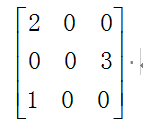若对其进行压缩存储，我们可以将一个非零数组元素的三元看成一个单位存入一维数组，具体如下所示。比如（1,1,1）代表第一行第一列的元素值为1。注意，这里我们只存储非零值。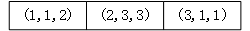具体代码如下：
#include<stdio.h>
#include <stdlib.h>
#include <time.h>
#define NUMBER  3

//三元组结构体
typedef struct {
//行标r，列标c
int r,c;
//元素值
int data;
}triple;
//矩阵的结构表示
typedef struct {
//存储该矩阵中所有非0元素的三元组
triple data[NUMBER];
//row和column分别记录矩阵的行数和列数，num记录矩阵中所有的非0元素的个数
int row,column,num;
}TSMatrix;
//输出存储的稀疏矩阵
void print(TSMatrix M);
int main() {
int i;
srand((unsigned)time(NULL));
TSMatrix M;
M.row=3;
M.column=3;
M.num=3;
//初始化矩阵
for(i=0;i<M.num;i++){
//随机数范围[1,3]
M.data[i].r=rand()%M.num+1;
M.data[i].c=rand()%M.num+1;
M.data[i].data=rand()%10;
}
print(M);
return 0;
}
void print(TSMatrix M){
for(int i=1;i<=M.row;i++){
for(int j=1;j<=M.column;j++){
int value =0;
for(int k=0;k<M.num;k++){
//遍历时的r,c行列值和实际存储row,column行列值的比较，相同的话就说明有非零元素,打印存储的非0值
if(i == M.data[k].r && j == M.data[k].c){
printf("%d ",M.data[k].data);
value =1;
break;
}
}
if(value == 0)
printf("0 ");
}
printf("\n");
}
}


行逻辑链接的顺序表
使用三元组顺序表存储稀疏矩阵，我们每次访问其中一个元素都要遍历整个矩阵，效率比较低。我们可以使用一个一维数组来存储每行第一个非零元素在一维数组中的位置，这样就可以提升访问效率。这样的表就叫做行逻辑链接的顺序表。
下图为一个稀疏矩阵，当使用行逻辑链接的顺序表对其进行压缩存储时，需要做以下两个工作：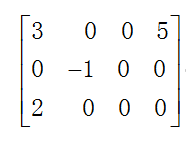1.将矩阵中的非 0 元素采用三元组的形式存储到一维数组 data 中：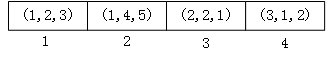2.使用数组 rpos 记录矩阵中每行第一个非 0 元素在一维数组中的存储位置。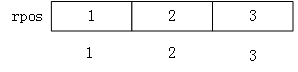通过以上两步操作，即实现了使用行逻辑链接的顺序表存储稀疏矩阵。
此时，如果想从行逻辑链接的顺序表中提取元素，则可以借助 rpos 数组提高遍历数组的效率。
例如，提取图 1 稀疏矩阵中的元素 2 的过程如下：
由 rpos 数组可知，第一行首个非 0 元素位于data，因此在遍历此行时，可以直接从第 data 的位置开始，一直遍历到下一行首个非 0 元素所在的位置（data）之前；
同样遍历第二行时，由 rpos 数组可知，此行首个非 0 元素位于 data，因此可以直接从第 data 开始，一直遍历到下一行首个非 0 元素所在的位置（data）之前；遍历第三行时，由 rpos 数组可知，此行首个非 0 元素位于 data，由于这是矩阵的最后一行，因此一直遍历到 rpos 数组结束即可（也就是 data[tu]，tu 指的是矩阵非 0 元素的总个数）。
具体代码如下

版权声明：本文为博主原创文章，遵循 CC 4.0 BY-SA 版权协议，转载请附上原文出处链接和本声明。
本文链接：https://blog.csdn.net/qq_16933601/article/details/106391152

#include <stdio.h>
#include <stdlib.h>
#include <time.h>
#define MAXSIZE 12500
#define MAXRC 100
typedef struct
{
//行，列
int r,c;
//元素值
int e;
}Triple;

typedef struct
{
//矩阵中元素的个数
Triple  data[MAXSIZE];
//每行第一个非零元素在data数组中的位置
int rpos[MAXRC];
//行数，列数，元素个数
int row,column,number;
}RLSMatrix;
//矩阵的输出函数
void print(RLSMatrix M){
for(int i=1;i<=M.row;i++){
for(int j=1;j<=M.column;j++){
int value=0;
if(i+1 <=M.row){
for(int k=M.rpos[i];k<M.rpos[i+1];k++){
if(i == M.data[k].r && j == M.data[k].c){
printf("%d ",M.data[k].e);
value=1;
break;
}
}
if(value==0){
printf("0 ");
}
}else{
for(int k=M.rpos[i];k<=M.number;k++){
if(i == M.data[k].r && j == M.data[k].c){
printf("%d ",M.data[k].e);
value=1;
break;
}

}
if(value==0){
printf("0 ");
}
}

}
printf("\n");
}
}
int main()
{
int i;
//srand((unsigned)time(NULL));

RLSMatrix M;
//矩阵的大小
M.number = 4;
M.row = 3;
M.column = 4;
//每一行首个非零元素在一维数组中的位置
M.rpos = 1;
M.rpos = 3;
M.rpos = 4;

M.data.e = 3;
M.data.r = 1;
M.data.c = 2;

M.data.e = 5;
M.data.r = 1;
M.data.c = 4;

M.data.e = 1;
M.data.r = 2;
M.data.c = 3;

M.data.e = 2;
M.data.r = 3;
M.data.c = 1;
//输出矩阵
print(M);
return 0;
}


十字链表法
关于十字链表法，具体可看下图。我们把矩阵的每一行每一列分别看成一个链表，然后将每一行和每一列的链表的第一个元素存放在一个数组中。这个数组就叫行链表的头指针数组，列链表的头指针数组。当我们访问矩阵的时候，就可以从行/列头指针数组中取出对应的指针，就可以访问这一行或者这一列的元素了。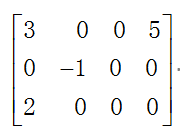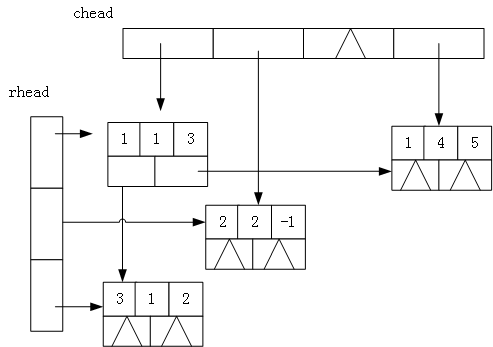链表中节点的结构应如下图。所以，除了定义三元组的行，列，数值外，我们还需要定义指向行的指针，指向列的指针。最后还需要定义一个存放行/列链表头结点的数组专门存放各行各列的头结点。具体代码如下。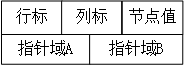typedef struct CLNode
{
//矩阵三元组i代表行 j代表列 e代表当前位置的数据
int r, c, data;
//指针域 行指针 列指针
struct CLNode *prow, *pcolumn;
typedef struct
{
//矩阵的行数,列数和非零元的个数
int rows, columns, num;
}CrossList;

下面我们将要根据用户输入的行数，列数，非零元素的值，来创建矩阵。
//注意检查用户的输入
do {
flag = 1;
printf("输入矩阵的行数、列数和非0元素个数：");
scanf("%d%d%d",&m,&n,&t);
if (m<0 || n<0 || t<0 || t>m*n)
flag = 0;
}while (!flag);
M.rows = m;
M.columns = n;
M.num = t;

用户输入合法的情况下我们要创建并初始化存放行列链表头的数组。
    //因为下标从1开始，所以头结点指针多分配一个内存
{
printf("初始化矩阵失败\r\n");
exit(0);
}
// 初始化行头指针向量;各行链表为空链表
for (r = 1; r <= m; r++)
{
}
// 初始化列头指针向量;各列链表为空链表
for (c = 1; c <= n; c++)
{
}

存放行列链表头的数组准备好了，接下来我们就要创建数据节点了。根据用户输入的行号，列好，数值创建节点。这里同样要检查用户的输入。
for (scanf("%d%d%d", &r,&c,&data); ((r<=0)||(c<=0)); scanf("%d%d%d", &r,&c,&data))
{
if (!(p = (CLNode*)malloc(sizeof(CLNode))))
{
printf("初始化三元组失败");
exit(0);
}
p->r = r;
p->c = c;
p->data= data;
}

当创建好一个节点之后，我们就要放到行或者列的正确的位置。根据输入的行号列号确定插入的位置。那么应该怎样去插入？分两种情况
1、当这一行中没有结点的时候，那么我们直接插入
2、当这一行中有结点的时候我们插入到正确的位置
对于第一个问题，因为之前已经对头结点数组进行了初始化NULL，所以直接根据NULL==M->rhead[i]就可以判断一行中有没有节点。
对于第二个问题，当行中有节点的时候，无非是插入到某个节点之前或者某个节点之后。什么时候插入到节点前？什么时候插入到节点后呢？
1.插入节点前：当我们要插入的节点的列号小于已经存在的节点的列号，这个时候就要插入到这个节点之前了。
2.插入节点后：当我们要插入的节点的列号大于已经存在的节点的列号，这个时候就要插入到这个节点之后了。
对于第一种情况，代码如下。
//p为准备插入的节点，要插入到M.rhead[r]之前。
{
}

对于第二种情况，我们要插入的节点插入到已有节点的后面，那么，已有将要插入节点的列号必定大于已有节点的列号。我们只要找到一个节点比我们将要插入节点的列号大的节点就好，然后插入到这个节点的前面。如果现有的结点没有一个结点列号是大于要插入的节点的列号的，那么我们就应该插入到最后一个结点之后！
              //我们要找到一个比q节点大的节点。在这个节点之前插入
for (q = M.rhead[r]; (q->prow) && q->prow->c < c; q = q->prow);
p->prow = q->prow;
q->prow = p;

对于列的插入同样如此，就不一一分析了，下面给出具体代码。
       //链接到列的指定位置
{
}
else
{
for (q = M.chead[c]; (q->pcolumn) && q->pcolumn->r < r; q = q->pcolumn);
p->pcolumn = q->pcolumn;
q->pcolumn = p;
}

打印矩阵
对于十字链表矩阵的打印，我们每次从行/列头结点数组中取出每一行或者每一列的第一个节点依次往下访问就可以了，和普通的链表访问没有区别。如果对链表不熟悉的可以参考这篇文章史上最全单链表的增删改查反转等操作汇总以及5种排序算法(C语言)
void PrintClist(CrossList M)
{
for (int i = 1; i <= M.num; i++)
{
{
while (NULL != p)
{
printf("%d\t%d\t%d\n",p->r, p->c, p->data);
p = p->pcolumn;
}
}
}
}

完整代码如下：
/*
* @Description: 十字链表存储压缩矩阵
* @Version: V1.0
* @Autor: Carlos
* @Date: 2020-05-26 16:43:48
* @LastEditors: Carlos
* @LastEditTime: 2020-05-28 14:40:19
*/
#include<stdio.h>
#include<stdlib.h>
typedef struct CLNode
{
//矩阵三元组i代表行 j代表列 e代表当前位置的数据
int r, c, data;
//指针域 行指针 列指针
struct CLNode *prow, *pcolumn;
typedef struct
{
//行和列链表头指针
//矩阵的行数,列数和非零元的个数
int rows, columns, num;
}CrossList;
CrossList InitClist(CrossList M)
{
CLNode *p,*q;
int r,c,data;
int m, n, t;
int flag;

//  printf("输入矩阵的行数、列数和非0元素个数：");
// scanf("%d%d%d",&m,&n,&t);
do {
flag = 1;
printf("输入矩阵的行数、列数和非0元素个数：");
scanf("%d%d%d",&m,&n,&t);
if (m<0 || n<0 || t<0 )
flag = 0;
}while (!flag);
M.rows = m;
M.columns = n;
M.num = t;
//因为下标从1开始，所以头结点指针多分配一个内存
{
printf("初始化矩阵失败\r\n");
exit(0);
}
// 初始化行头指针向量;各行链表为空链表
for (r = 1; r <= m; r++)
{
}
// 初始化列头指针向量;各列链表为空链表
for (c = 1; c <= n; c++)
{
}
//行数列数不为0
for (scanf("%d%d%d", &r,&c,&data); ((r<=0)||(c<=0)); scanf("%d%d%d", &r,&c,&data))
{
if (!(p = (CLNode*)malloc(sizeof(CLNode))))
{
printf("初始化三元组失败");
exit(0);
}
p->r = r;
p->c = c;
p->data= data;
//链接到行的指定位置。
{
}
else
{
for (q = M.rhead[r]; (q->prow) && q->prow->c < c; q = q->prow);
p->prow = q->prow;
q->prow = p;
}
//链接到列的指定位置
{
}
else
{
for (q = M.chead[c]; (q->pcolumn) && q->pcolumn->r < r; q = q->pcolumn);
p->pcolumn = q->pcolumn;
q->pcolumn = p;
}

}
return M;
}

void PrintClist(CrossList M)
{
for (int i = 1; i <= M.num; i++)
{
{
while (NULL != p)
{
printf("%d\t%d\t%d\n",p->r, p->c, p->data);
p = p->pcolumn;
}
}
}
}
int main()
{
CrossList M;
M = InitClist(M);
PrintClist(M);
return 0;
}

文中代码均已测试，有任何意见或者建议均可联系我。欢迎学习交流！
如果觉得写的不错，请点个赞再走，谢谢！


展开全文数据结构与算法 十字链表
• 在介绍矩阵的压缩存储前，我们需要明确一个概念：对于特殊矩阵，比如对称矩阵，稀疏矩阵，上（三角矩阵，在数据结构中相同的数据元素只存储一个。 @[TOC] 三元组顺序表 稀疏矩阵由于其自身的稀疏特性，通过压缩...
• 诀窍是通过输出(它们的行)在下三角形中查找值 . 您可以为每行的数据保留一个索引，因为您行顺序访问输入的行顺序时的条目 .与博览会类型struct CSR { // sometimes implicitly symmetricstd::vector vals;std::...
• 3.4特殊矩阵和压缩矩阵 类比数组： 一维数组： 二维数组，采用映射的方法：按行优先和按列优先 行优先： 列优先： ...（对称矩阵、上（三角矩阵、对角矩阵） 对称矩阵 ：若一个n阶方阵A[1…n][1...
• 一、概念 严版数据结构：特殊矩阵：相同元素或者零元素在矩阵中的分布...特殊矩阵存储这里，上、下三角矩阵存储。对称矩阵是角对角线对称。 由对称矩阵A[i][j] = A[j][i]，所以我们只需要保存一份即可，所需...
• ## 矩阵

2006-07-18 14:18:00
矩阵的顺序表示 按行优先顺序存储: loc(aij) = loc(a00) + i x n +j 按列优先顺序存储: loc(aij) = loc(a00) + jx m +i 下三角矩阵存储 : loc(aij) = loc(a00) + i x (i+1)/2 +j (0≤j≤i≤n-1) 伪地址表示法...
• 文章目录数组数组的存储结构一维数组的存储结构二维数组的存储结构一、二维数组按行优先存放二、二维数组按列优先存放特殊矩阵的压缩存储一、对称矩阵的压缩存储二、上、下三角矩阵的压缩存储上三角矩阵下三角矩阵三...数据结构 算法
• （2）三角矩阵：分为上三角矩阵，下三角矩阵 例题：假设有一个n*n的三角矩阵，第一个元素为a,请用一种存储效率高的形式将其存储在一维数组中 （3）对角矩阵 此处过，考的不多，考了就pass掉做别的题目 例题：
• 按列优先 特殊矩阵的压缩 特殊矩阵： • 对称矩阵 • 上（三角矩阵 • 对角矩阵 对称矩阵 规律：ai,j = a j,i n*n 矩阵 压缩：存在一维数组中（也可以存储三角矩阵） 第一行： 1个元素 a1,1 第二行： 2个...
• 矩阵存在数组中两种方式：按行存储按列存数(地址公式不记，脑海构思自己想) 压缩矩阵：相同的元素压缩到一个数组单元中，0元素不存储 特殊举证的压缩存储： 对称矩阵 ->只存上三角 or 下三角 三角举证 ->只...数据结构
• 数组的存储结构：一维数组、多维数组都是存放在一个按行优先(也可以按列优先)的一维长数组中。 特殊矩阵(方阵)的压缩存储 对称矩阵(symmetric matrix) 对称矩阵中的元素是按主对角线对称的，即上三角部分和下三角...
• 按行优先、按列优先 2、矩阵 为了节省空间，找出矩阵中相同元素的分布规律进行压缩 对称矩阵 新的元素下标为 k={i(i−1)2+j−1i≥j(下三角区和主对角元素)j(j−1)2+i−1i<j(上三角区元素aij=aji) k=\left\{ \...数据结构 算法
• 图的存储结构除了要存储 图中各个顶点本身的信息，还要存储边的信息。 常见的图的存储结构有邻接矩阵和邻接表。 邻接矩阵是表示顶点之间相邻关系的矩阵。...局限性：要确定图中有多少条边，必须按行，按列对每个元素
• 一、邻接矩阵1、描述：用一维数组存储图顶点的信息用二维数组存储图边的信息2、特点：①无向图的邻接矩阵：是唯一的对称矩阵，可以压缩存储（仅存储上/下三角）；第i行（）非零元素为第i个顶点的度②有向图的邻接...
• 数组和广义表 1. 数组 一维数组 二维数组： ...普通矩阵的存储：二维数组的存储 ...描述矩阵元素时，行、号通常从1开始，而描述数组时通常...下三角矩阵：除了对角线和下三角区，其余元素都相同 行优先存入一维数组数据结构 数组
•  按列存储 a+(i+j*m) *len 稀疏矩阵： 如果一个n*n矩阵里大量的元素都是0的话，则称之为稀疏矩阵  1.上三角矩阵 ：矩阵中下标为i,j的元素对应的一维数组下标计算方式(2n-i+1)*i/2+j  2.下三角矩阵软件设计师
• 可以使用按行或按列运算来确定缩小的下三角矩阵L的元素，并证明它们是高斯​​枢轴行乘数的置换乘积之和。 这些排列积的总和可以使用树结构构造，该树结构可以容易地存储或使用矩阵积计算。 与LU分解中元素的存储...
• 2．对称矩阵的压缩存储：以行序为主序存储下三角中的元素，包括对角线上的元素。二维下标为( i, j ),存储空间的一维下标为k，给出k与 i, j (i)的关系k=（ ） （1, j , 0*(n+1)/2)。 A．i*(i-1)/2+j-1 B．i*(i+1)/2+j...
• 20.假设一10阶下三角矩阵按列优顺序压缩存储一维数组C中，则C数组的大小为________。 21.在n个结点的二叉链表中，有________个空指针。 22.对关键字序列(52，80，63，44，48，21)进行一趟简单选择排序之后得到的结果...数据结构题
• 选中D1单元格，用鼠标指向单元格右角的小方块“■”，当光标变成"＋"后，按住鼠标拖动光标向拖到要合并的结尾行处，就完成了B和C的合并。这时先不要忙着把B和C删除，先要把D的结果复制一下，再用...excel
• 矩阵按键扫描程序是一种节省IO口的方法,按键数目越多节省IO口就越可观，思路：先判断某一（行）是否有按键按下，再判断该行（）是那一只键按下。但是，在程序的写法上，采用了最简单的方法，使得程序效率最高。...
• 9.8将一个5×5的矩阵中最大的元素放在中心，4个角分别放在4个最小的元素（从左到右，从上到的顺序，依次从小到大存放），写一个函数实现之，并用main函数调用。 78 10.9在主函数中输入10个等长的字符串。用另一...
• 实例154　继承层次对类排序 实例155　查看内部类信息 7.2　反射的进阶 实例156　动态设置类的私有域 实例157　动态调用类中的方法 实例158　动态实例化类 实例159　创建长度可变的数组 实例160　利用反射重写...Java
• 实例154　继承层次对类排序 实例155　查看内部类信息 7.2　反射的进阶 实例156　动态设置类的私有域 实例157　动态调用类中的方法 实例158　动态实例化类 实例159　创建长度可变的数组 实例160　利用反射重写...Java
• 实例154　继承层次对类排序 实例155　查看内部类信息 7.2　反射的进阶 实例156　动态设置类的私有域 实例157　动态调用类中的方法 实例158　动态实例化类 实例159　创建长度可变的数组 实例160　利用反射重写...Java